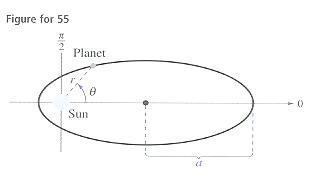# Polar Form of the Equation of a Conic

## Homework Statement

The planets travel in an elliptical orbit with the sun as a focus. Assume that the focus is at the pole, the major axis lies on the polar axis and the length of the major axis is 2a. Show that the polar equation of orbit is given by $$r=\frac{(1-e^2)a}{1-e\cos\theta}$$

here's a diagram they give:## The Attempt at a Solution

Ok, I have converted the equation of an ellipse in rectangular form: $$\frac{x^2}{a^2}+\frac{y^2}{b^2}=1$$

to polar form before, yielding $$r^2=\frac{b^2}{1-e^2\cos^2\theta}$$

this seems like it would be a similar process.

On the standard form of the ellipse in rectangular form, I believe you would have (x+c)2 because with one focus at the origin the center is going to be c away from the focus (I know It's plus c because they give a diagram showing the center in the positive x axis; rather than negative which would be minus c)

so would you be converting

$$\frac{(x+c)^2}{a^2}+\frac{y^2}{b^2}=1$$

to polar form to yield the equation given?

$$\frac{(r\cos\theta+c)^2}{a^2}+\frac{(r\sin\theta)^2}{b^2}=1$$

$$a^2(r^2\cos^2\theta+2cr\cos\theta+c^2)+b^2(r^2\sin^2\theta)=a^2b^2$$

Before I go any deeper am I on the right path? Thanks.

#### Attachments

Last edited:

lanedance
Homework Helper
it may help to know some useful properties of ellipses, the eccentcity is defined as
$$e = \sqrt{1-\frac{b^2}{a^2}}$$

and the distance from the focus to the centre of the ellipse is
$$f = ae$$